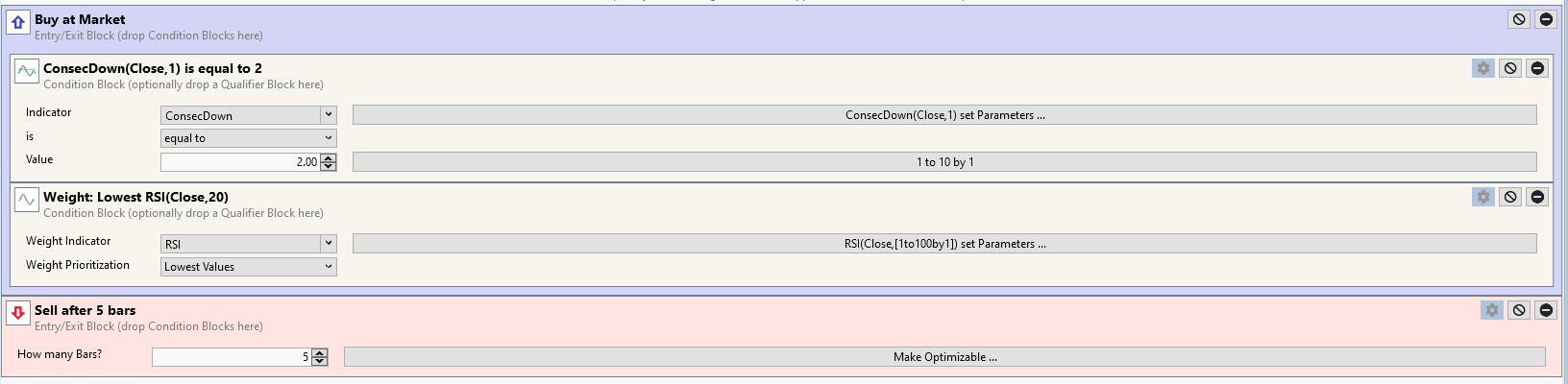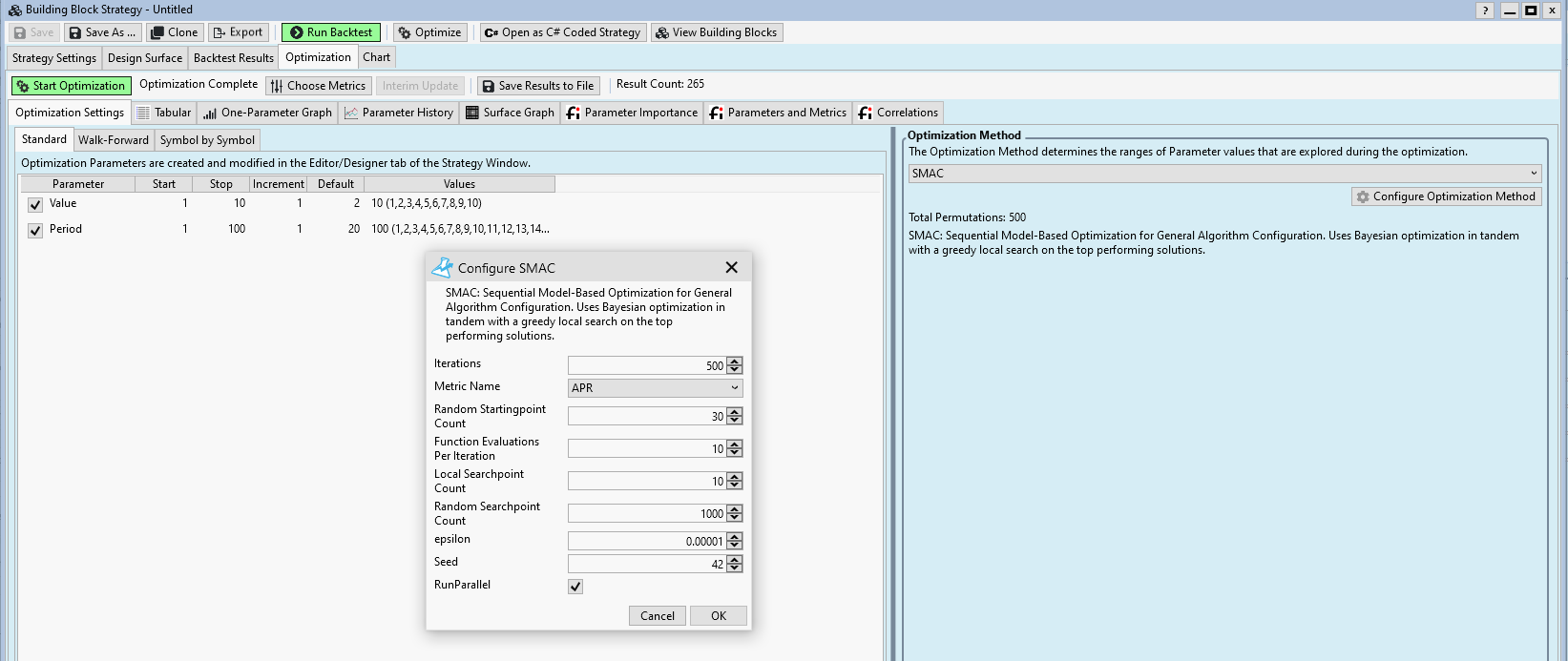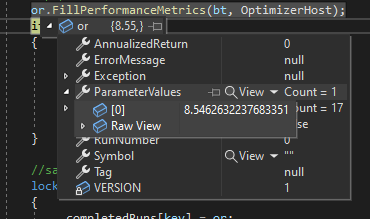Hello,
if you use the ConsecDown compares to value in a Building Blocks strategy, the SMAC Optimizer returns 0 APR for all parameter pairs.
Even if the ConsecDown is used as an additional block.1
77
Solved
8 Replies

Bookmark

Sort
If you look at the C# code of your strategy you'll find:

```CODE: public MyStrategy() : base()
{
AddParameter("Value", ParameterType.Double, 1, 1, 5, 1);
...
}```

This means: The "Value" parameter is implemented as a double parameter. (While the ConseqDown indicator returns just integer values)

And later:

```CODE:if (indicator[index] == Parameters.AsDouble)
{
condition0 = true;
...```

Here is a equality comparison between two double values ...
... this is a big sin in computer science: never compare doubles for equality.

The problem gets bigger when you use one of the finantic Optimizers. These optimizers use the Min/Max values of parameters only, the step value is ignored.

The problem gets obscured by the Tabular result window which in turn shows the "Value" parameter as a integer (which it is not).

Solution
Don't compare the value of ConseqDown with an "equal to" operator. Use "greater than or equal to" instead.

I think the "equal to" operator should be flagged with some "use with care" label which might explain that an "equal to" operation between an indicator and some double value probably never hits.
1
This isn’t the issue DrKoch, because this works fine in other optimizers like Exhaustive. The issue is that SMAC does not adhere to the discrete indicator values, but chooses values between the discreet stepping stones.
0
Here we see in debug mode that SMAC assign 8.54 to the parameter value, so this will never be equal to this indicator's return values, which always have zero decimal places. And, all indicators are expressed as TimeSeries, a range of floating-point double, so there's really no way to return integers here. Maybe SMAC can be given an option to round its values to the nearest whole number?
00
QUOTE:
Maybe SMAC can be given an option to round its values to the nearest whole number?

All finantic Optimizers do this if the ParameterType is Int32.
0
Yes but this is a case of it being a double. And like i said that’s not changeable since it’s an Indicator Compare to Value (Value is always double even though this particular indicator only ever returns whole numbers.)
0
Probably I can do a dirty trick and cosider a parameter as Integer valued if min and max and step have integer values.
0
Hmm that sounds error prone, would almost rather see a concrete setting exposed, but maybe it would work?
0